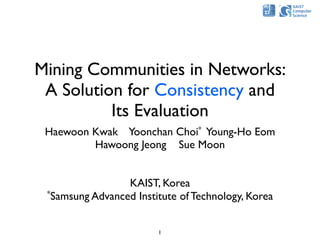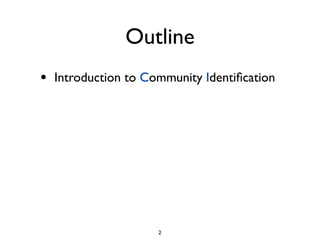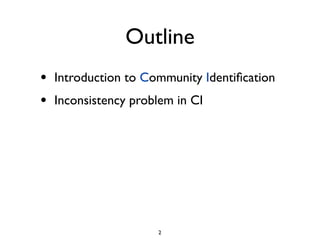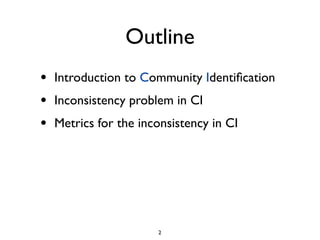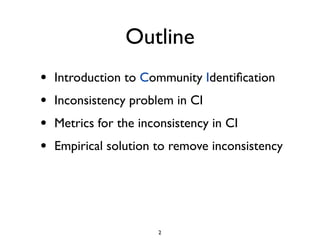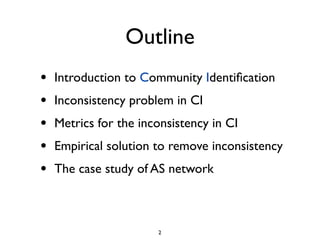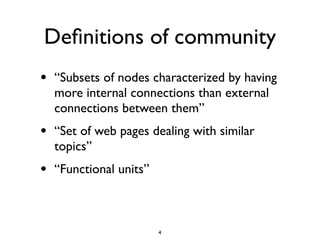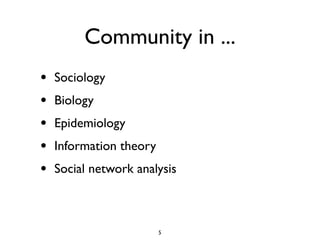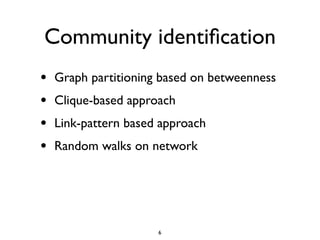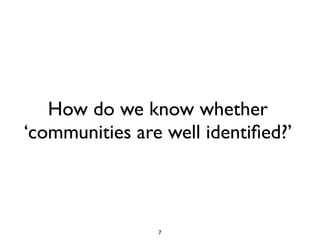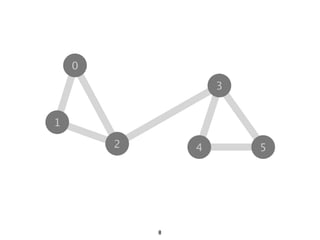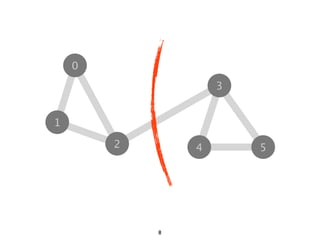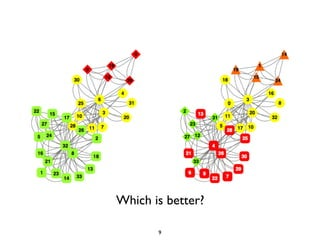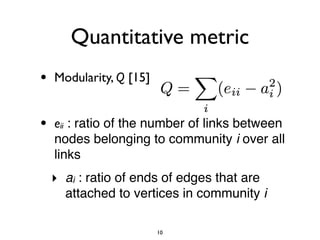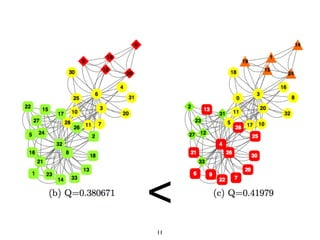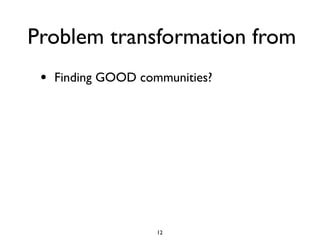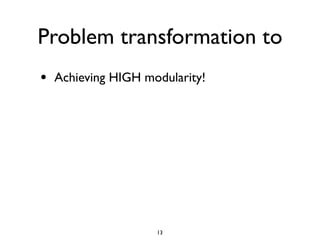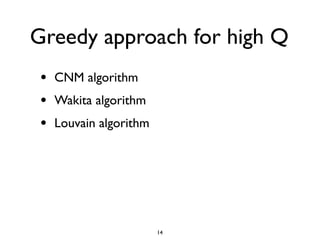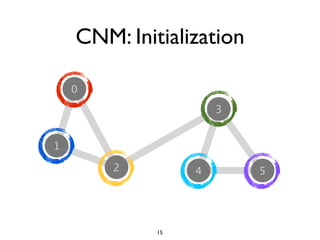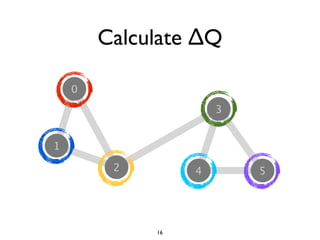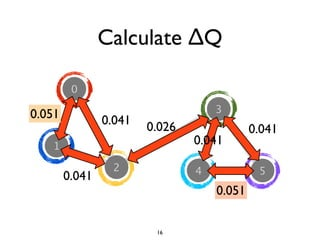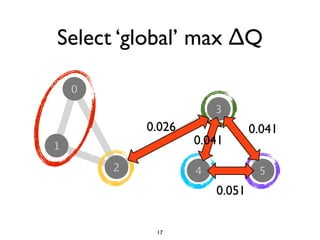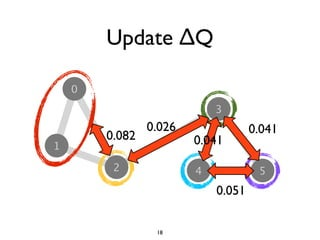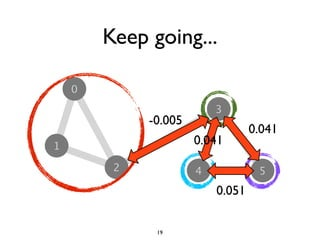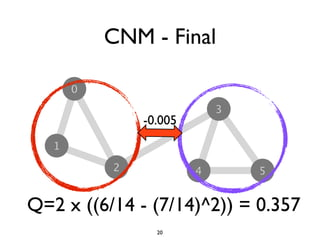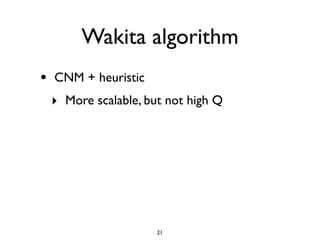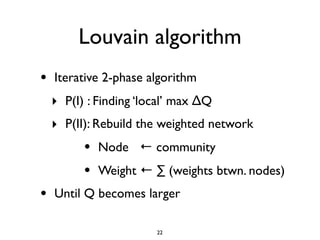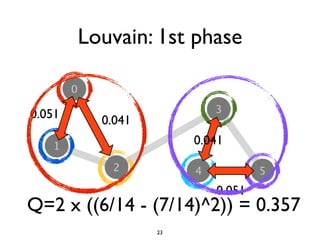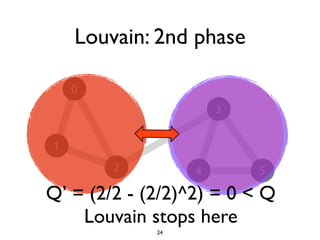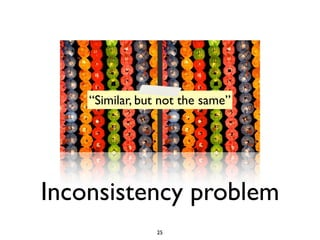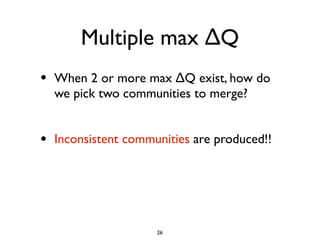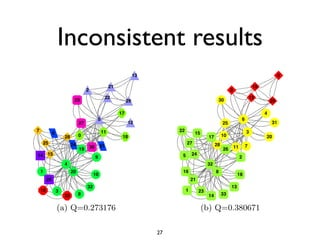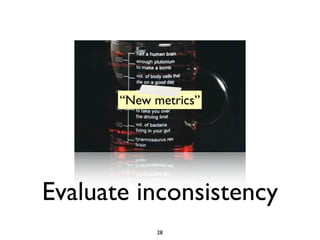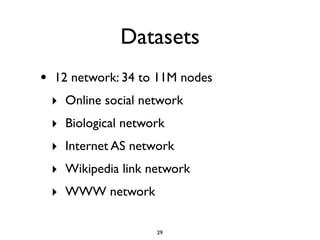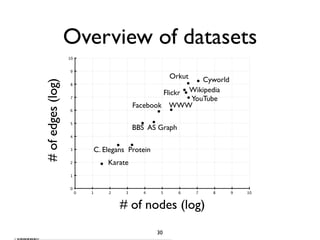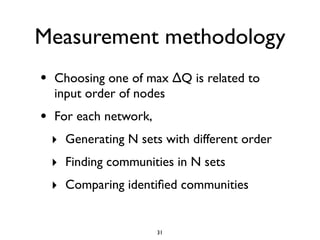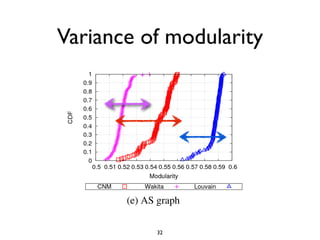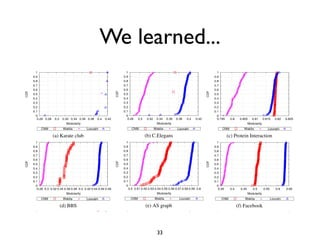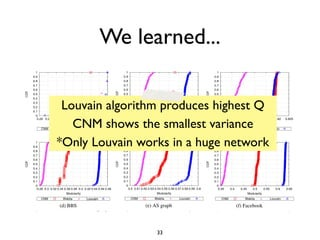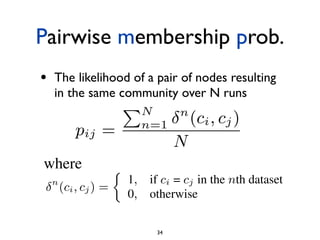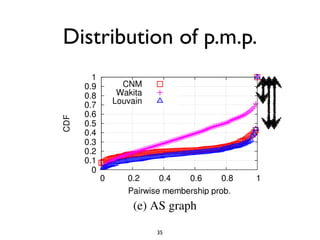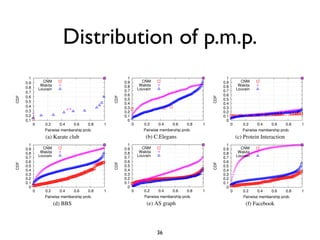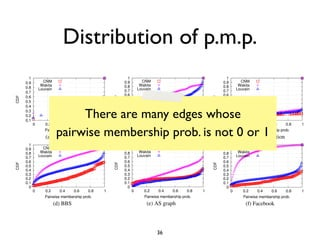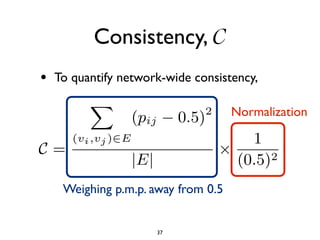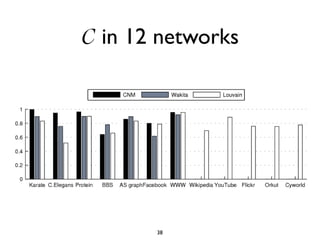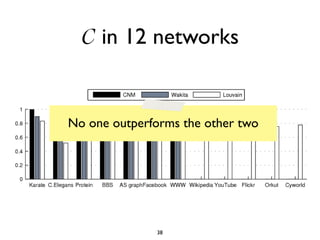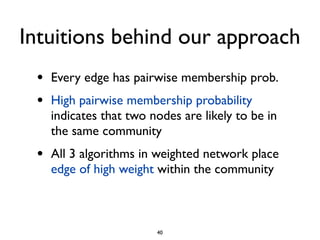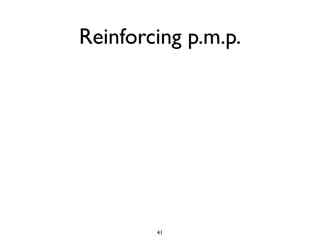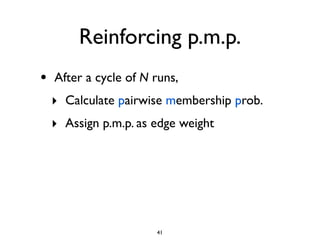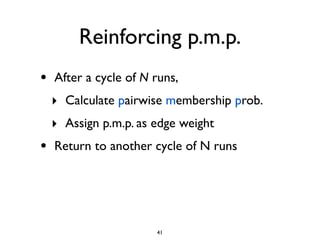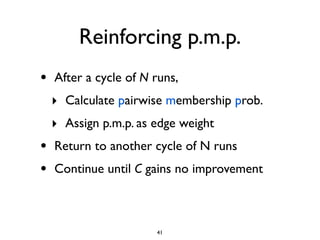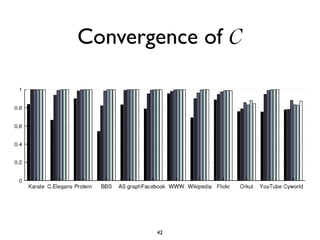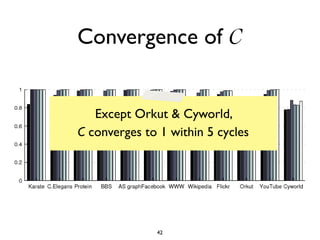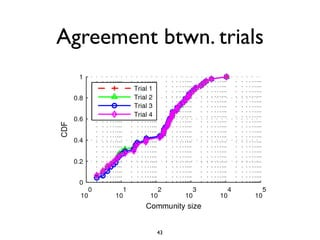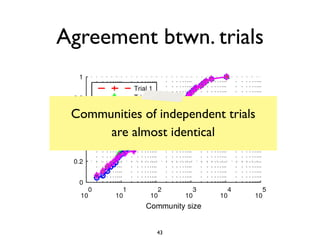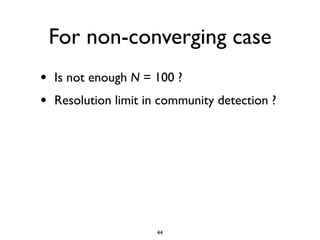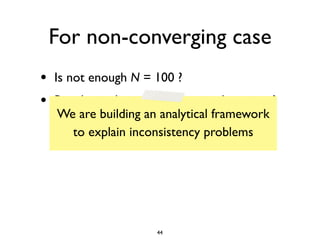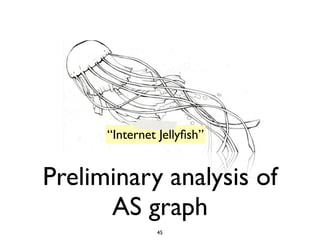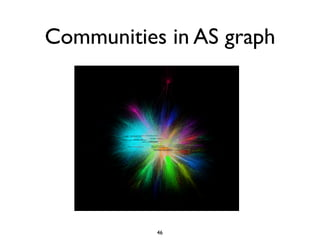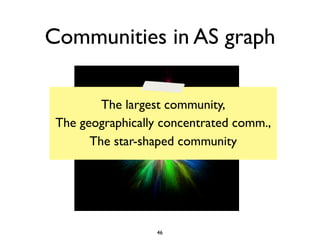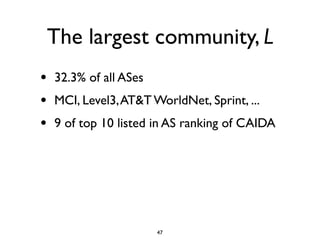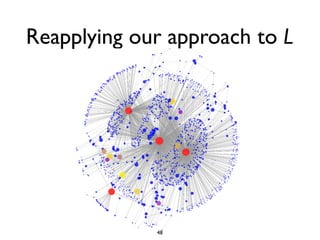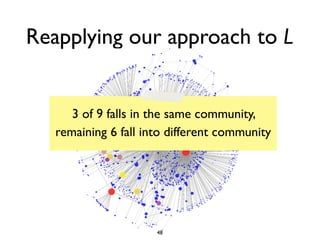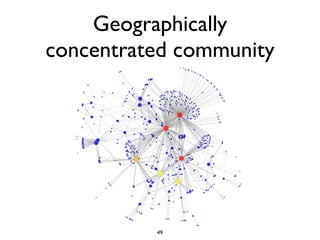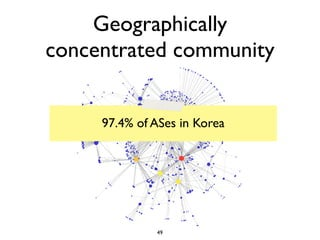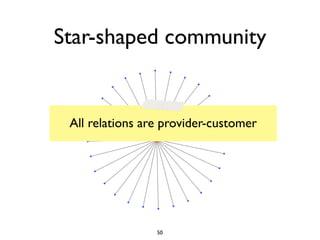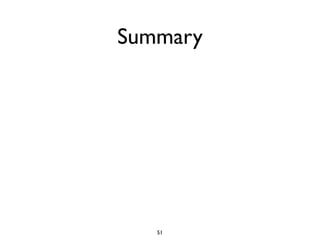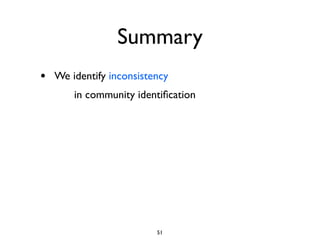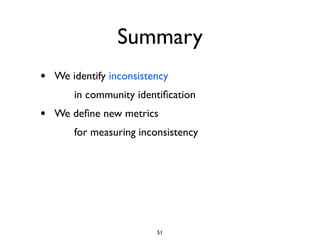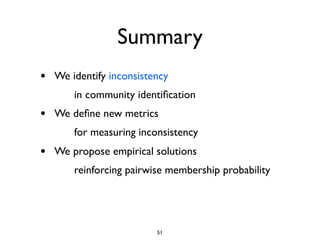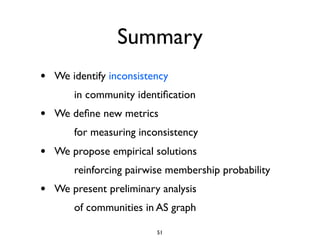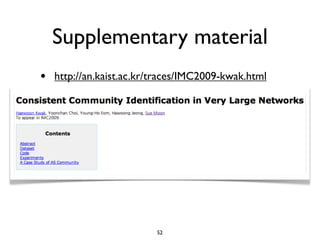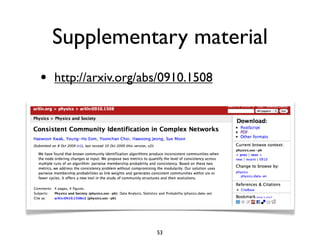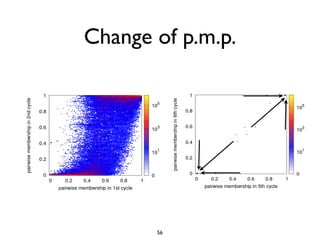1 of 79

### Mining Communities in Networks: A Solution for Consistency and Its Evaluation

1. Mining Communities in Networks: A Solution for Consistency and Its Evaluation Haewoon Kwak Yoonchan Choi* Young-Ho Eom Hawoong Jeong Sue Moon KAIST, Korea *Samsung Advanced Institute of Technology, Korea 1
2. Outline 2
3. Outline • Introduction to Community Identiﬁcation 2
4. Outline • Introduction to Community Identiﬁcation • Inconsistency problem in CI 2
5. Outline • Introduction to Community Identiﬁcation • Inconsistency problem in CI • Metrics for the inconsistency in CI 2
6. Outline • Introduction to Community Identiﬁcation • Inconsistency problem in CI • Metrics for the inconsistency in CI • Empirical solution to remove inconsistency 2
7. Outline • Introduction to Community Identiﬁcation • Inconsistency problem in CI • Metrics for the inconsistency in CI • Empirical solution to remove inconsistency • The case study of AS network 2
8. “Sense of Community” Introduction 3
9. Deﬁnitions of community • “Subsets of nodes characterized by having more internal connections than external connections between them” • “Set of web pages dealing with similar topics” • “Functional units” 4
10. Community in ... • Sociology • Biology • Epidemiology • Information theory • Social network analysis 5
11. Community identiﬁcation • Graph partitioning based on betweenness • Clique-based approach • Link-pattern based approach • Random walks on network 6
12. How do we know whether ‘communities are well identiﬁed?’ 7
13. 8
14. 8
15. Which is better? 9
16. Quantitative metric • Modularity, Q  • eii : ratio of the number of links between nodes belonging to community i over all links ‣ ai : ratio of ends of edges that are attached to vertices in community i 10
17. < 11
18. Problem transformation from • Finding GOOD communities? 12
19. Problem transformation to • Achieving HIGH modularity! 13
20. Greedy approach for high Q • CNM algorithm • Wakita algorithm • Louvain algorithm 14
21. CNM: Initialization 15
22. Calculate ∆Q ‣ 16
23. Calculate ∆Q ‣ 0.051 0.041 0.026 0.041 0.041 0.041 0.051 16
24. Select ‘global’ max ∆Q ‣ 0.026 0.041 0.041 0.051 17
25. Update ∆Q ‣ 0.026 0.041 0.082 0.041 0.051 18
26. Keep going... ‣ -0.005 0.041 0.041 0.051 19
27. CNM - Final ‣ -0.005 Q=2 x ((6/14 - (7/14)^2)) = 0.357 20
28. Wakita algorithm • CNM + heuristic ‣ More scalable, but not high Q 21
29. Louvain algorithm • Iterative 2-phase algorithm ‣ P(I) : Finding ‘local’ max ∆Q ‣ P(II): Rebuild the weighted network • Node ← community • Weight ← ∑ (weights btwn. nodes) • Until Q becomes larger 22
30. Louvain: 1st phase ‣ 0.051 0.041 0.041 0.051 Q=2 x ((6/14 - (7/14)^2)) = 0.357 23
31. Louvain: 2nd phase ‣ Q’ = (2/2 - (2/2)^2) = 0 < Q Louvain stops here 24
32. “Similar, but not the same” Inconsistency problem 25
33. Multiple max ∆Q • When 2 or more max ∆Q exist, how do we pick two communities to merge? • Inconsistent communities are produced!! 26
34. Inconsistent results 13 0 21 19 2 9 22 12 23 29 30 29 17 4 5 6 27 12 25 31 7 11 22 3 6 0 15 10 28 18 17 20 25 24 27 28 30 31 11 7 19 26 14 15 9 5 24 2 4 32 1 20 16 8 10 18 26 21 32 13 16 3 1 23 33 8 14 33 (a) Q=0.273176 (b) Q=0.380671 FIG. 1: [Color Online] Visualization of inconsistent community identiﬁcation in the K 27 belong to the same community, and node ordering is depicted as the number in the nod
35. “New metrics” Evaluate inconsistency 28
36. Datasets • 12 network: 34 to 11M nodes ‣ Online social network ‣ Biological network ‣ Internet AS network ‣ Wikipedia link network ‣ WWW network 29
37. Overview of datasets 10 9 Orkut Cyworld # of edges (log) 8 Flickr Wikipedia 7 YouTube Facebook WWW 6 5 BBS AS Graph 4 3 C. Elegans Protein 2 Karate 1 0 0 1 2 3 4 5 6 7 8 9 10 # of nodes (log) 30
38. Measurement methodology • Choosing one of max ∆Q is related to input order of nodes • For each network, ‣ Generating N sets with different order ‣ Finding communities in N sets ‣ Comparing identiﬁed communities 31
39. Variance of modularity b (b) C.Elegans (c) Prot (e) AS graph (f) 32
40. We learned... (a) Karate club (b) C.Elegans (c) Protein Interaction (d) BBS (e) AS graph (f) Facebook 33
41. We learned... Louvain algorithm produces highest Q CNM shows the smallest variance (a) Karate club (b) C.Elegans (c) Protein Interaction *Only Louvain works in a huge network (d) BBS (e) AS graph (f) Facebook 33
42. Figure 6: Consistency (no data available Pairwise membershiprandomly orde runs of an algorithm, each over a prob. Over runs of an algorithm, each over a ra uantify set, we an algorithm, eachpaira of nodespair of the likelihood of alikelihood of aordered inp Over• The likelihood of athe of nodes resulting runs of quantify pair over randomly resulti munity as: community as: aover of runs resulting in t set, we quantify same community pair N nodes same the likelihood of in the same community as: ( where where 1 if = in the th dataset 1 1 the th dataset 0if otherwise in = if = in the 0 otherwise 0 otherwise and and are nodes and and represent communities that and belong to, respectively. 34We call this metric pairwise mem and and are nodes and and represe
43. Distribution of p.m.p. (b) C.Elegans (e) AS graph 35
44. Distribution of p.m.p. (a) Karate club (b) C.Elegans (c) Protein Interaction (d) BBS (e) AS graph (f) Facebook 36
45. Distribution of p.m.p. There are many edges whose pairwise membership prob. is not (c) Protein Interaction (a) Karate club (b) C.Elegans 0 or 1 (d) BBS (e) AS graph (f) Facebook 36
46. ms produce pairwise membership probabilities of ’s. For the remaining nine networks, Louvain p Consistency, C t consistent outcome and, for (g) to (h), the only ou der to quantify network-wide community members , we deﬁne a metric of consistency for the entire • To quantify network-wide consistency, Normalization sistency Weighing p.m.p. pairwise 0.5 weighs the away from membership prob om . The second term in (4) normalizes from e of communities detected by CNM algorithm in th 37
47. C in 12 networks Figure 6: Consistency (no data available by CNM and Wakita for Wikipedia and Cyworld) ver runs of an algorithm, each over a randomly ordered input we quantify the likelihood of a pair of nodes resulting in the 38
48. C in 12 networks No one outperforms the other two Figure 6: Consistency (no data available by CNM and Wakita for Wikipedia and Cyworld) ver runs of an algorithm, each over a randomly ordered input we quantify the likelihood of a pair of nodes resulting in the 38
49. “Totally intuitive” Our approach 39
50. Intuitions behind our approach • Every edge has pairwise membership prob. • High pairwise membership probability indicates that two nodes are likely to be in the same community • All 3 algorithms in weighted network place edge of high weight within the community 40
51. Reinforcing p.m.p. 41
52. Reinforcing p.m.p. • After a cycle of N runs, ‣ Calculate pairwise membership prob. ‣ Assign p.m.p. as edge weight 41
53. Reinforcing p.m.p. • After a cycle of N runs, ‣ Calculate pairwise membership prob. ‣ Assign p.m.p. as edge weight • Return to another cycle of N runs 41
54. Reinforcing p.m.p. • After a cycle of N runs, ‣ Calculate pairwise membership prob. ‣ Assign p.m.p. as edge weight • Return to another cycle of N runs • Continue until C gains no improvement 41
55. Convergence of C Figure 8: Convergence of consistency erforms the other two in all networks and no consistent correla- 42 between the consistency and the topological characteristics of
56. Convergence of C Except Orkut & Cyworld, C converges to 1 within 5 cycles Figure 8: Convergence of consistency erforms the other two in all networks and no consistent correla- 42 between the consistency and the topological characteristics of
57. Agreement btwn. trials nvergence of consistency la- of us- FI- the all all hip is, Figure 10: Comparison of community size distribution in 4 tri- 43
58. Agreement btwn. trials nvergence of consistency la- of us- Communities of independent trials FI- are almost identical the all all hip is, Figure 10: Comparison of community size distribution in 4 tri- 43
59. For non-converging case • Is not enough N = 100 ? • Resolution limit in community detection ? 44
60. For non-converging case • Is not enough N = 100 ? • Resolution limit in community detection ? We are building an analytical framework to explain inconsistency problems 44
61. “Internet Jellyﬁsh” Preliminary analysis of AS graph 45
62. Communities in AS graph 46
63. Communities in AS graph The largest community, The geographically concentrated comm., The star-shaped community 46
64. The largest community, L • 32.3% of all ASes • MCI, Level3, AT&T WorldNet, Sprint, ... • 9 of top 10 listed in AS ranking of CAIDA 47
65. Reapplying our approach to L 48
66. Reapplying our approach to L 3 of 9 falls in the same community, remaining 6 fall into different community 48
67. Geographically concentrated community 49
68. Geographically concentrated community 97.4% of ASes in Korea 49
69. Star-shaped community All relations are provider-customer 50
70. Summary 51
71. Summary • We identify inconsistency in community identiﬁcation 51
72. Summary • We identify inconsistency in community identiﬁcation • We deﬁne new metrics for measuring inconsistency 51
73. Summary • We identify inconsistency in community identiﬁcation • We deﬁne new metrics for measuring inconsistency • We propose empirical solutions reinforcing pairwise membership probability 51
74. Summary • We identify inconsistency in community identiﬁcation • We deﬁne new metrics for measuring inconsistency • We propose empirical solutions reinforcing pairwise membership probability • We present preliminary analysis of communities in AS graph 51
75. Supplementary material • http://an.kaist.ac.kr/traces/IMC2009-kwak.html 52
76. Supplementary material • http://arxiv.org/abs/0910.1508 53
77. Thank you 54
78. Backup slides 55

### Editor's Notes

1. Hi, I&amp;#x2019;m Haewoon Kwak, a ph. d student of KAIST, Korea. Today I&amp;#x2019;m gonna talk about inconsistency problem in community identification and its empirical solution. This work is collaboration with ...
2. If there are many methods to find communities in network,
3. If a network becomes more complex, we are not sure which partitioning is better
4. The modularity, Q, is a quality measure of partitioned communities. For each community i, we calculate the difference between the fraction of the number of within-community edges and the square of the fraction of the sum of degrees over all links. The value of modularity ranges from -1 to 1. The value Q = 1 is the maximum, indicates strong community structure
5. Obtaining the highest modularity is NP-hard problem, so approximation algorithms are used.
6. The CNM algorithm begins with each node as a separate community in a network
7. Then the algorithm finds the pair of communities with the global maximum &amp;#x394;Q. Community pairs of maximum gain of modularity. In this example there are two maximum delta Q. Algorithm choose one of them according to implementation
8. Then the algorithm finds the pair of communities with the global maximum &amp;#x394;Q. Community pairs of maximum gain of modularity. In this example there are two maximum delta Q. Algorithm choose one of them according to implementation
9. Then the algorithm finds the pair of communities with the global maximum &amp;#x394;Q. Community pairs of maximum gain of modularity. In this example there are two maximum delta Q. Algorithm choose one of them according to implementation
10. Then the algorithm finds the pair of communities with the global maximum &amp;#x394;Q. Community pairs of maximum gain of modularity. In this example there are two maximum delta Q. Algorithm choose one of them according to implementation
11. Then the algorithm finds the pair of communities with the global maximum &amp;#x394;Q. Community pairs of maximum gain of modularity. In this example there are two maximum delta Q. Algorithm choose one of them according to implementation
12. Then the algorithm finds the pair of communities with the global maximum &amp;#x394;Q. Community pairs of maximum gain of modularity. In this example there are two maximum delta Q. Algorithm choose one of them according to implementation
13. Then the algorithm finds the pair of communities with the global maximum &amp;#x394;Q. Community pairs of maximum gain of modularity. In this example there are two maximum delta Q. Algorithm choose one of them according to implementation
14. Then the algorithm finds the pair of communities with the global maximum &amp;#x394;Q. Community pairs of maximum gain of modularity. In this example there are two maximum delta Q. Algorithm choose one of them according to implementation
15. Then the algorithm finds the pair of communities with the global maximum &amp;#x394;Q. Community pairs of maximum gain of modularity. In this example there are two maximum delta Q. Algorithm choose one of them according to implementation
16. Then the algorithm finds the pair of communities with the global maximum &amp;#x394;Q. Community pairs of maximum gain of modularity. In this example there are two maximum delta Q. Algorithm choose one of them according to implementation
17. Then the algorithm finds the pair of communities with the global maximum &amp;#x394;Q. Community pairs of maximum gain of modularity. In this example there are two maximum delta Q. Algorithm choose one of them according to implementation
18. Then the algorithm finds the pair of communities with the global maximum &amp;#x394;Q. Community pairs of maximum gain of modularity. In this example there are two maximum delta Q. Algorithm choose one of them according to implementation
19. Then the algorithm finds the pair of communities with the global maximum &amp;#x394;Q. Community pairs of maximum gain of modularity. In this example there are two maximum delta Q. Algorithm choose one of them according to implementation
20. Then the algorithm finds the pair of communities with the global maximum &amp;#x394;Q. Community pairs of maximum gain of modularity. In this example there are two maximum delta Q. Algorithm choose one of them according to implementation
21. updates &amp;#x394;Q values that correspond to any neighboring community of the newly merged community
22. In the first phase, it starts with single-node communities like CNM &amp; Wakita. Each node is moved to the adjacent community that maximize delta Q. If delta Q is negative, the node stays at original community. In the second phase, the algorithm re- builds the network with /communities as nodes/ and /sum of weights between nodes as link weights/, and returns to the first phase.
23. So far we have presented the process of three algorithms.
24. Some of you feel unclear about a part of algorithm, choosing one of maximum delta Q. now we move on to the problem of inconsistency
25. From this Figure, there is a great difference between two partitioning. In left, 7 communities are identified, and in right only three communities identified. This network has only 34 nodes. Thus, if network becomes larger, we can predict the problems become more serious. In next section, we quantitatively show the significance of inconsistent problems.
26. Now we move on measuring inconsistency.
27. AS Graph is from work by Oliveira,&quot;Quantifying the Completeness of the Observed Internet AS-level Structure&quot; Social network data except Cyworld is from work by Alan mislove and Meeyoung cha.
28. First, we compare the distribution of modularity. You remember modularity is the quality measure of partitioning. From the distribution of modularity, we know how different partitioning is.
29. The pairwise membership probability represents the empirical probability that two nodes belong to the same community across multiple runs of the same algorithm. If two nodes are always in the same community, the value becomes 1 or, two nodes are always in the different community, the value is 0 We consider pairwise membership probability only between neighbors.
30. The larger the proportion of 0 or 1 is, the more consistent the communities are
31. No one algorithm outperforms the other two in all networks and no correlation between out consistency and the topological characteristics of a network, such as average degree, link density, and average clustering coefficient.
32. We discuss plausible reasons later
33. This plots the community size distributions from independent trials. All 4 plots almost completely overlap and are very close to each other.
34. Our choice of N = 100 is to make sure that we break ties in choosing max delta Q, but 100 might be not large enough to break all possible ties in Cyworld and Orkut. / Fortunato and Barth&amp;#xE9;lemy report that communities below a certain size may not be resolved and are grouped into a larger loose community. The resolution limit is the threshold community size, and is a function of the total number of links, not nodes.
35. So far we have seen how to identify communities in a consistent manner. Now we need to check whether identified communities are meaningful. Here we apply consistent community identification to AS graph.
36. Out of 48 communities, we found interesting communities: the largest community, a geographically concentrated community, and a star-shaped community
37. The layers of strongly connected tier-1 ASes at the core and other tier-1 ASes remind us of the Internet Jellyfish model. We leave in-depth mapping of our communities to the jellyfish model for future work
38. Next, we draw geographically concentrated community. For manual inspection, we choose the community with top Korean ISPs. This community has 658 ASes, and 97.4% of ASes are in Korea. HK! The interesting point is physical constratins such as transatlantic and pacific lines somehow manifest thru grouping.
39. We found a star-shaped community. All leaf ASes connect only to the hub AS and no other. They are single- homed stub ASes. One notable observation is that in this community of a star topology there is no peer-peer relation
40. This is preliminary. We found many interesting things for future work.
41. This is preliminary. We found many interesting things for future work.
42. This is preliminary. We found many interesting things for future work.
43. This is preliminary. We found many interesting things for future work.
44. We color the 100 by 100 grids according to the number of links with the corresponding pairwise member- ship probabilities in two consecutive cycles.